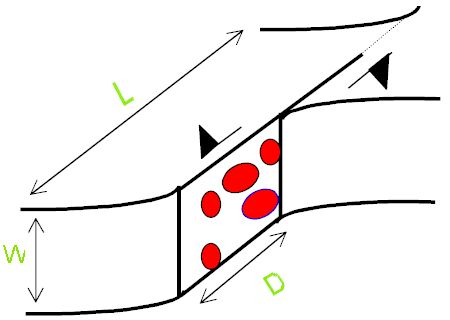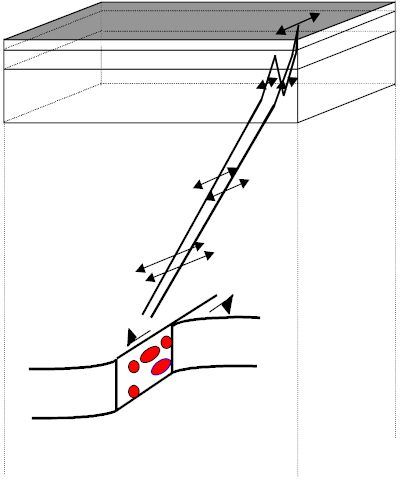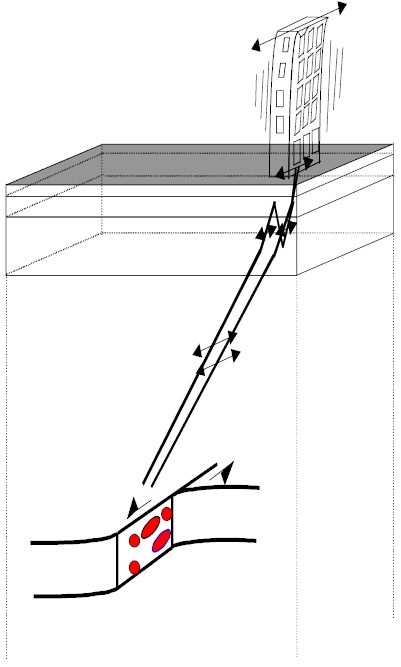# Concepts

Seismic Source

Earthquakes in a classical sense how to write a analysis essay originate from the rapid release of elastic energy caused by the rupture of rock in the earth. Damaging effects due to an earthquake arise (i) from a source outcropping directly at the earths surface, (ii) from the radiated seismic waves. In practice the second effect is the most important. It occurs more frequently and the area shattered by seismic waves is by far larger than the zone which could be hit by an outcropping source. In ShakyGround we focus, therefore, on the effects caused by the radiation of seismic waves. The simulation of the seismic source in ShakyGround follows a stochastic concept as it has been proposed by Hanks & McGuire (1981) and Boore (1983). The source signal, in terms of ground acceleration, is generated as a sequence of gaussian distributed random numbers. The general characteristics of the seismic source are represented by applying a window function in the time domain and filtering the signal with respect to the spectral properties of the seismic source. The concept thus reflects the high variability typically in most accelerograms, which can be reconducted to heterogeneities of the source (the so-called ‘barriers’ or ‘asperities’, schematically indicated by the red spots in the figure). On the other hand, the deterministic frame parameters, like source dimension (represented here by the length L and the width W) and the average dislocation D, are accounted for by the window function and the spectral filtering.Wave Propagation

Its now commonly accepted that wave propagation effects have a strong effect on strong ground motion. On their way to the surface, seismic waves undergo modifications due to reflection, refraction and absorption. We account for these effects by the use of Haskell propagator matrices and a complex wave number approach for the absorption. Horizontally polarized S-waves (SH-waves) are considered, since these are the most important ones for earthquake engineering purposes. The geological structure is represented by a sequence of horizontally layered plane strata overlying an infinite halfspace, which contains the seismic source. The seismic signal is strongly affected by the subsurface geological structure. Considerable amplitude amplifications occur if the velocity (and density) of the uppermost layers are lower than the velocities (and densities) of the material below. Thicknesses and velocities of the uppermost layers are typically in a range that makes amplitude amplification effects occur at frequencies between 1 and 20 Hz., i. e., the frequency interval of technical do my homework math interest. The sketch displays the modifations the seismic signal undergoes on its way from the source to the receiver. Amplitudes are supposed to be large close to the source, then they decrease as the wave travels towards the surface. Within the layer stack the signal is refracted and reflected. In the layers close to the surface, which we suppose to have a low impedance, we observe amplitude amplification.Structure Response

A simple and widely used approach for the representation of the structure response is the so-called “response spectrum” method. Response spectra form the basis of seismic regulation codes, such as the USAEC, US building code in the United States and the EC 8 in Europe. In this context the structure is supposed to behave like a simple oscillator, such as a spring or pendulum. The characteristic parameters of this model are the natural frequency and the damping, which are determined by size, configuration and material makeup of the structure. Earthquake ground motion will excite the building and cause it to “resonate” according to its preferred frequency. The calculation of response spectra consists of several steps. First, select the natural period and damping, then calculate the relative displacement of the oscillator, i. e. its “response” with respect to ground, and note the maximum amplitude of the response. This is repeated for a set of natural frequencies in order to get a “spectrum” of responses. A dangerous situation arises if the natural frequency of a structure falls in the frequency range of major seismic radiation. In this case the accelerations in the structure may be several times higher than the peak ground acceleration measured at the surface.